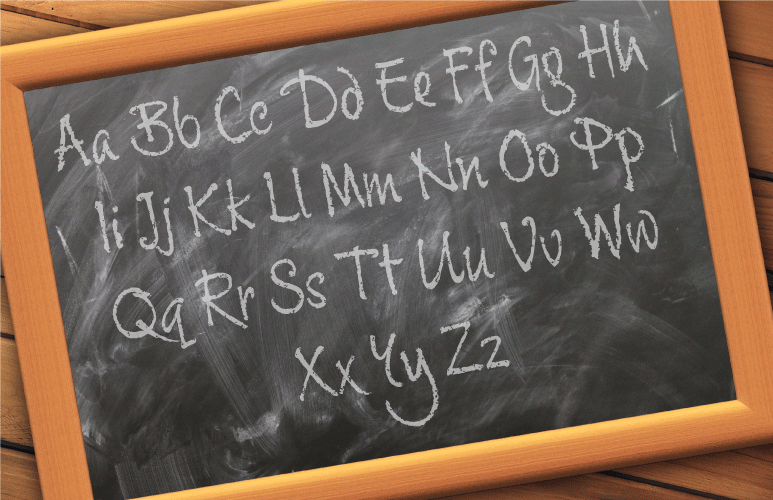Not everyone will need to do this lesson, but if the English Alphabet is completely new to you, you might need to practise actually writing the letters.

### Learn

#### The English alphabet

There are 26 letters in the English alphabet. They can be written LARGE (in capitals) or small (in small letters).

Watch and listen:-

Letter Listen How to form the letter Letter name
A Capital A: Draw an angled vertical line facing right: /. Draw another angled vertical line facing left: \, both lines should touch at the top: /\. Draw a horizontal line in the middle of the two lines -.
This is A.
ai
B Capital B: Draw a vertical line: | . On its right side, draw two half-bubbles, going down the line. This is B. bee
C

Capital C: Draw a half-moon, with an opening on the right.
This is C.

see
D Capital D: Draw a vertical line: |. Then, starting at its top right, draw a backwards C (step 3).
This is D.
dee
E Capital E: Draw one vertical line: |. Draw three horizontal lines -, on the right side of this, each 1/3rd shorter than the original (but the middle line is shorter than the lines on the top and bottom). One goes on top, one in the middle, one on bottom.
This is E.
ee
F

Capital F: Draw an E (step 5), but omit the bottom horizontal line.
This is F.

ef
G Capital G: Draw a C. Then, draw a horizontal line -, beginning at the base of the bottom tip, half-way through the C.
This is G.
gee
H Capital H: Draw two vertical lines next to each other: | |. Then, draw a horizontal line in the middle, connecting them -.
This is H.
aitch
I Capital I: Draw one vertical line: |.
This is I.
eye
J Capital J: Draw an I with a hook on it. Like a backward-facing fish hook.
This is J.
jay
K Capital K: Draw a vertical line: |. Then, draw two lines, starting from the right hand side, each from the middle. One angles up, the other down.
This is K.
kay
L Capital L: Draw a vertical line: |. Then, draw a shorter, horizontal line: _ on the bottom right.
This is L.
el
M Capital M: Draw two vertical lines next to another: | |. Then, starting from the inner, top tips, draw two shorter angled lines that touch 1/2 way in the middle.
This is M.
em
N Capital N: Draw two horizontal lines close to each other: | |. Then, draw an angled line: \ that starts from the inner top tip of the left line, angled so that it touches the other line, on the inner bottom tip.
This is N.
en
O Capital O: Draw a full circle.
This is O.
oh
P Capital P: Draw a vertical line: |. Then, draw a 1/2 bubble on the right side upper tip, and touching the middle of the vertical line: P.
This is P.
pee
Q Capital Q: Draw a full circle: O. Then, on the near-bottom right, draw a vertical line angling right, partly in the O, and partly out of it.
This is Q.
cue
R Capital R: Draw a P. Then, starting from where the bottom 1/2 bubble touches the vertical line, draw a small line angled to the right.
This is R.
ar
S Capital S: In one stroke, draw a wavy line going left, then right, then left.
This is S.
es
T Capital T: Draw a vertical line: |. Then, draw a shorter, horizontal line: _ on top.
This is T.
tee
U Capital U: Draw a circle, but, leave part of the top open.
This is U.
you
V Capital V: Draw two vertical lines next to each other, but, angle the left one to the right and down: \, and the right one to the left and down: /.
This is V.
vee
W

Capital W: Draw two V's next to another: W.
This is W.

double-you
X Capital X: Draw one vertical line headed up and right /. Draw another vertical line up and leaning left: \, crossing in the middle.
This is X.
ex
Y Capital Y: Draw a small v. Then, where the two lines meet, draw a vertical line | .
This is Y.
wi
Z Capital Z: In one stroke, draw a horizontal line:_, then a vertical line that angles downward left /, then a horizontal line to the right _.
This is Z.

zed in British English

zee in American English

##### Listen to Lynne as she says (and sings) the alphabet again. If you feel brave have a go at singing / saying the alphabet yourself.

###### !Note - All posts are moderated and then corrected. One correction per person per lesson.

Wouldn't it be cool if there were a teacher available here 24/7? Well, you could help us achieve our dream, and support us in updating and improving these resources by making a donation:-Created